# NCERT Solutions for Class 8 Maths Chapter 7 Cube and Cube Roots Ex 7.2

These NCERT Solutions for Class 8 Maths Chapter 7 Cube and Cube Roots Ex 7.2 Questions and Answers are prepared by our highly skilled subject experts.

## NCERT Solutions for Class 8 Maths Chapter 7 Cube and Cube Roots Exercise 7.2

Question 1.
Find the cube root of each of the following numbers by prime factorisation method.
(i) 64
(ii) 512
(iii) 10648
(iv) 27000
(v) 15625
(vi) 13824
(vii) 110592
(viii) 46656
(ix) 175616
(x) 91125
Solution:
(i) 64
On grouping the factors in triplets, we get
64 = 23 × 23
64 = (2 × 2)3 = 43
$$\sqrt{64}$$ = 4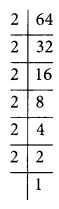(ii) 512
On grouping the factors in triplets, we get
512 = 23 × 23 × 23
= (2 × 2 × 2)3
= 83
$$\sqrt{512}$$ = 8(iii) 10648
On grouping the factors in triplets, we get
10648 = 23 × 113
= (2 × 11)3
= 223
$$\sqrt{10648}$$ = 22(iv) 27000
On grouping the factors in triplets, we get
27000 = 23 × 33 × 53
= (2 × 3 × 5)3
= 303
$$\sqrt{27000}$$ = 30(v) 15625
On grouping the factors in triplets, we get
15625 = 53 × 53
= (5 × 5)3
= 253
$$\sqrt{15625}$$ = 25(vi) 13824
On grouping the factors in triplets, we get
13824 = 23 × 23 × 23 × 33
= (2 × 2 × 2 × 3)3
= 243
$$\sqrt{13824}$$ = 24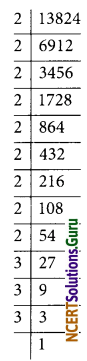(vii) 110592
On grouping the factors in triplets, we get
110592 = 23 × 23 × 23 × 23 × 33
= (2 × 2 × 2 × 2 × 3)3
= 483
$$\sqrt{110592}$$ = 48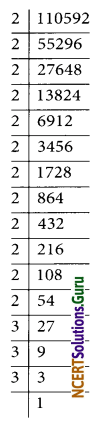(viii) 46656
On grouping the factors in triplets, we get
46656 = 23 × 23 × 33 × 33
= (2 × 2 × 3 × 3)3
= 363
$$\sqrt{46656}$$ = 36(ix) 175616
On grouping the factors in triplets, we get
175616 = 23 × 23 × 23 × 73
= (2 × 2 × 2 × 7)3
= 563
$$\sqrt{175616}$$ = 56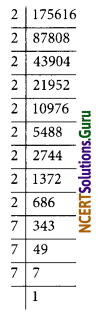(x) 91125
On grouping the factors in triplets, we get
91125 = 33 × 33 × 53
= (3 × 3 × 5)3
= 453
$$\sqrt{91125}$$ = 45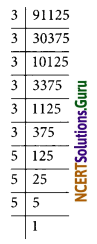Question 2.
State true or false.
(i) Cube of any odd number is even.
(ii) A perfect cube does not end with two zeros.
(iii) If the square of a number ends with 5, then its cube ends with 25.
(iv) There is no perfect cube which ends with 8.
(v) The cube of a two-digit number may be a three-digit number.
(vi) The cube of a two-digit number may have seven or more digits.
(vii) The cube of a single-digit number may be a single-digit number.
Solution:
(i) False
(ii) True
(iii) False [152 = 225; 153 = 3375]
(iv) False [123 = 1728]
(v) False [103 = 1000]
(vi) False [993 = 970299]
(vii) True [23 = 8]

Question 3.
You are told that 1,331 is a perfect cube. Can you guess without factorization what is its cube root? Similarly, guess the cube roots of 4913, 12167, 32768.
Solution:
(i) Separating the given number 1331 into two groups.
1 and 331
331 ends in 1
unit digit of the cube root = 1
Tens digit of the cube root = 1
$$\sqrt{1331}$$ = 11(ii) Cube root of 4913
Separating the given number 4913 in two groups i.e. 4 and 913
In this case, 913 has three-digit and 4 has only one digit
The digit 3 is at its own place. We take the one’s place of the required cube root as 7.
Take the other group i.e. 4, a cube of 1 is 1, and a cube of 2 is 8. 4 lies between 1 and 8.
The smaller number among 1 and 2 is 1
The one place of 1 is 1 itself.
Take 1 as ten’s place of the cube root of 4913.
$$\sqrt{4913}$$ = 17

(iii) Cube root of 12167
Separating 12167 in two groups i.e. 12 and 167
The digit 7 is at its one’s place. We take the one’s place of required cube root as 3.
The unit digit of the cube root = 3
Take the other group i.e. 12 Cube of 2 is 8 and cube of 3 is 27. 12 lies between 8 and 27
The smaller among 2 and 3 is 2
The one place is 2 itself.
Take 2 as ten’s place of the cube root of 12167.
$$\sqrt{12167}$$ = 23(iv) Cube root of 32768
Separating 32768 in two groups i.e. 32 and 768
Take 768
The digit 8 is at its one’s place so, the one’s place of the required cube root is 2.
Take the other group i.e. 32
The cube of 3 is 27 and the cube of 4 is 64.
32 lies between 27 and 64.
The smaller number between 3 and 4 is 3
Take 3 as ten’s place of the cube root of 32768
$$\sqrt{32768}$$ = 32

error: Content is protected !!